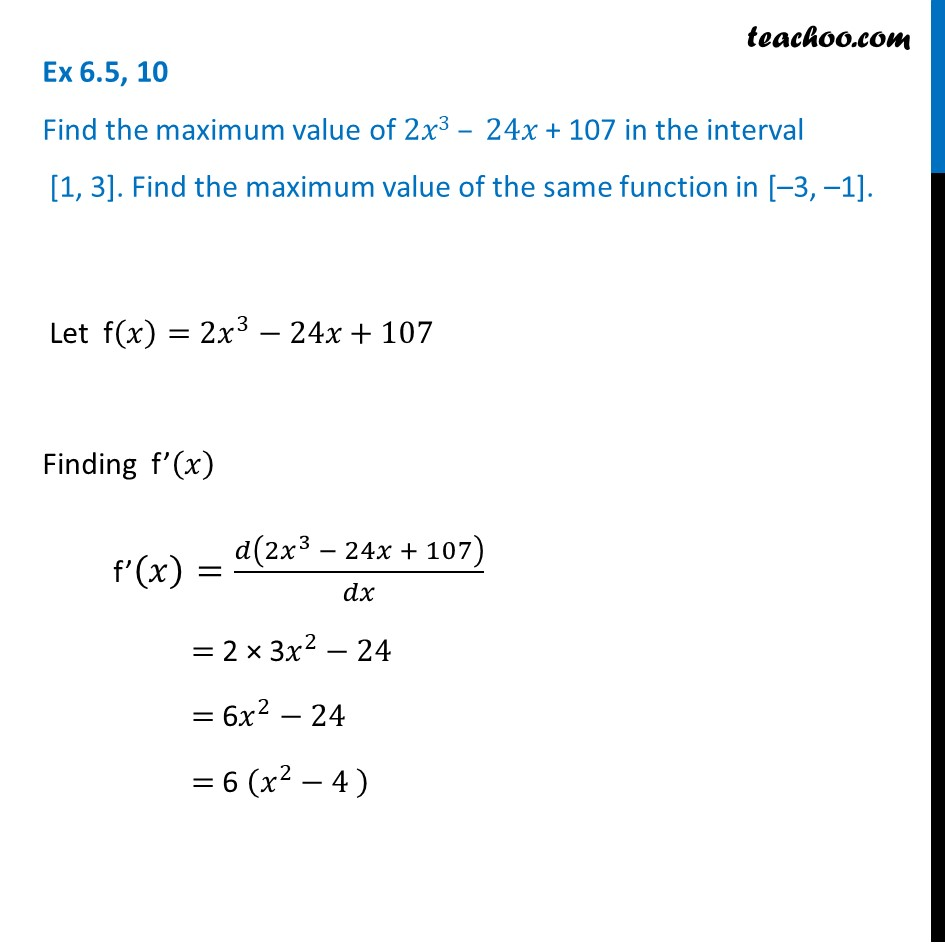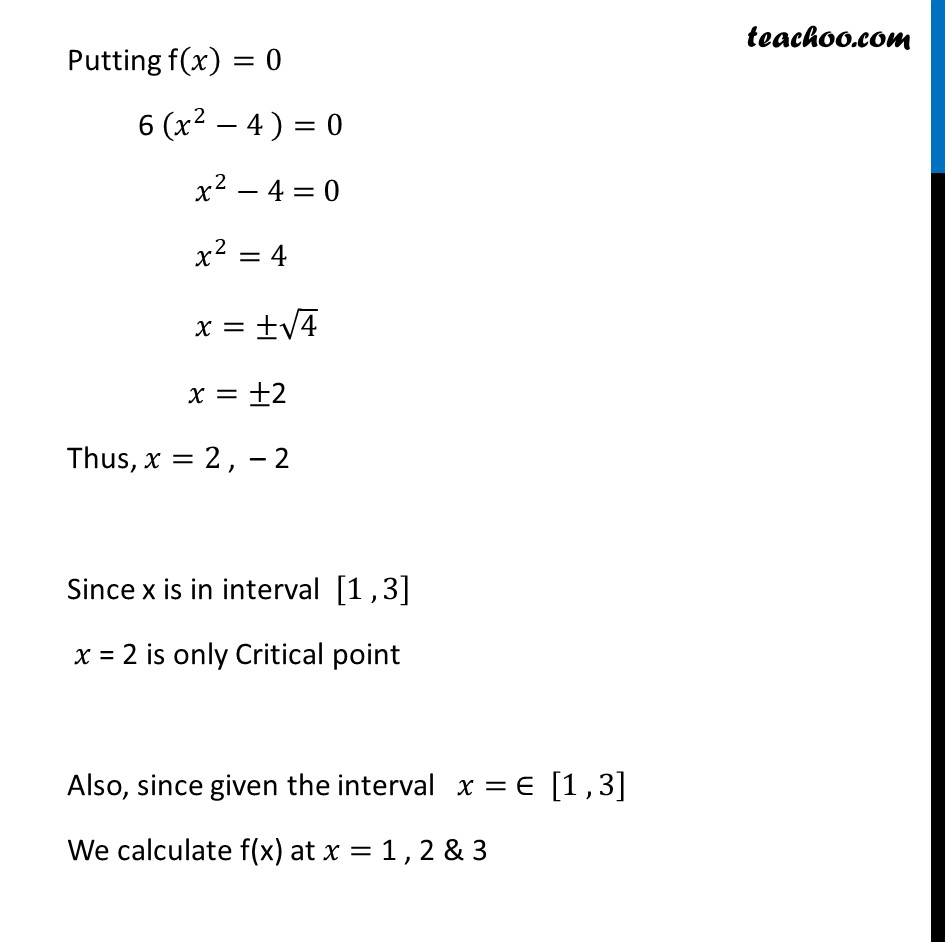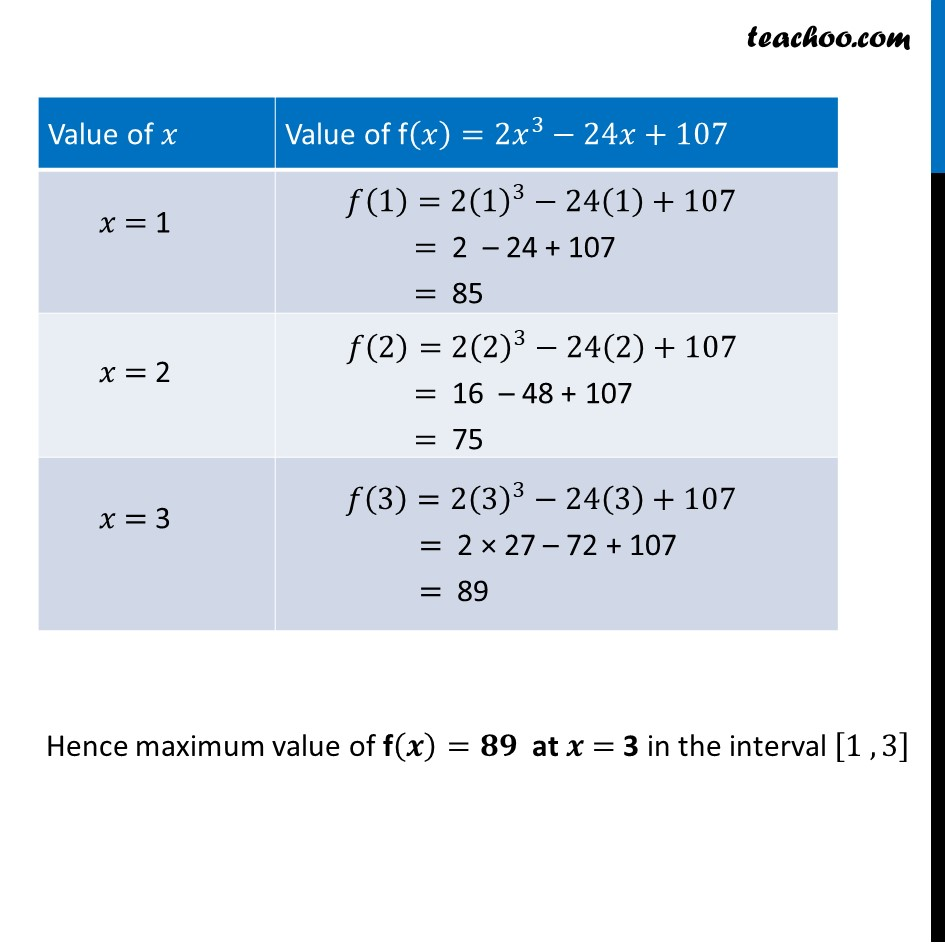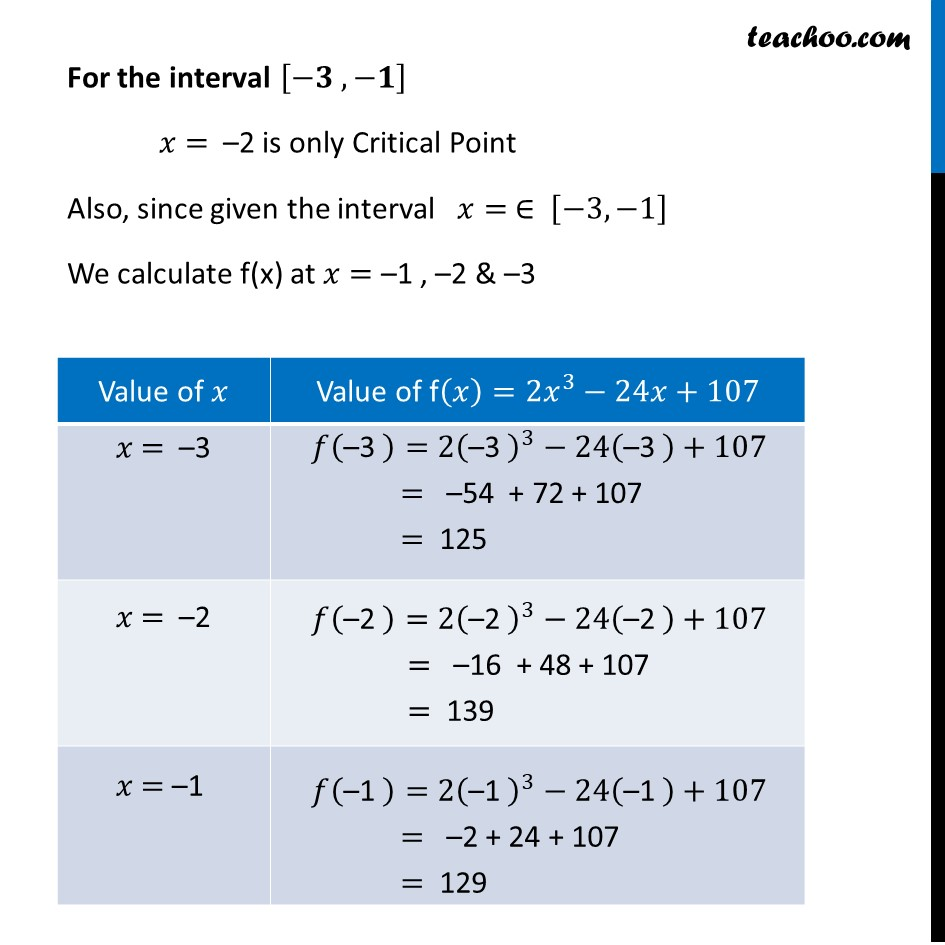Ex 6.3

Chapter 6 Class 12 Application of Derivatives
Serial order wiseLearn in your speed, with individual attention - Teachoo Maths 1-on-1 Class

### Transcript

Ex 6.3, 10 Find the maximum value of 2𝑥3 – 24𝑥 + 107 in the interval [1, 3]. Find the maximum value of the same function in [–3, –1]. Let f(𝑥)=2𝑥^3−24𝑥+107 Finding f’(𝑥) f’(𝑥)=𝑑(2𝑥^3 − 24𝑥 + 107)/𝑑𝑥 = 2 × 3𝑥^2−24 = 6𝑥^2−24 = 6 (𝑥^2−4" " ) Putting f(𝑥)=0 6 (𝑥^2−4" " )=0 𝑥^2−4" = 0 " 𝑥^2=4 𝑥=±√4 𝑥=±2 Thus, 𝑥=2 , – 2 Since x is in interval [1 , 3] 𝑥 = 2 is only Critical point Also, since given the interval 𝑥= ∈ [1 , 3] We calculate f(x) at 𝑥= 1 , 2 & 3 Hence maximum value of f(𝒙)=𝟖𝟗 at 𝒙 = 3 in the interval [1 , 3] For the interval [−𝟑 , −𝟏] 𝑥 = –2 is only Critical Point Also, since given the interval 𝑥= ∈ [−3,−1] We calculate f(x) at 𝑥= –1 , –2 & –3 Hence maximum value of f(𝒙)=𝟏𝟑𝟗 at 𝒙 = –2 in the interval [−3,−1]